﻿ WiFi外辐射源雷达参考信号重构及其对探测性能影响研究
«上一篇文章快速检索 高级检索

 雷达学报2016, Vol. 5Issue (3): 284-292  DOI: 10.12000/JR151080

### 引用本文 [复制中英文]

[复制中文]
Rao Yunhua, Ming Yanzhen, Lin Jing, et al. Reference signal reconstruction and its impact on detection performance of wiFi-based passive radar[J]. Journal of Radars, 2016, 5(3): 284-292. DOI: 10.12000/JR15108.
[复制英文]

### 文章历史

WiFi外辐射源雷达参考信号重构及其对探测性能影响研究

(武汉大学电子信息学院 武汉 430072)

Reference Signal Reconstruction and Its Impact on Detection Performance of WiFi-based Passive Radar

(Electronics Information School, Wuhan University, Wuhan 430072, China)
Foundation Items: National Natural Science Foundation of China (61271400, 41106156)
Abstract: While Wireless Fidelity (WiFi)-based passive radar can achieve high detection resolution in both the range and Doppler domain, it is difficult to extract the reference signal because of the complexities of its signal format and application scenarios. In this study, we analyze a typical application of WiFi-based passive radar and discuss different methods for reference signal extraction. Based on the format and features of WiFi signals, we propose a method for reference signal reconstruction, and analyze the influence of the reconstructed reference signal's performance on detection. The results show that higher reference SNRs generate lower decoding bit rate errors and better clutter suppression with the reconstructed reference signal. Moreover, we propose a method for removing irrelevant signals to avoid the impact on target detection of a non-direct path signal in the receiving signal. The experimental results validate the efficacy of the proposed signal processing method.
Key words: Wireless Fidelity (WiFi)     Passive Radar     Reference signal     Reconstruction

1 引言

2 典型WiFi信号模型

IEEE 802.11标准包括a/b/g/n等一系列协议，其中802.11g以其高速率与后向兼容性成为第3代WLAN标准，IEEE工作组为这一标准定义了DSSS/CCK(Complementary Code Keying)和OFDM等4种物理层。本文将以典型的OFDM模式进行分析，该模式下信号带宽可达20 MHz，具有较好的距离分辨率，其物理层帧结构如图 1所示。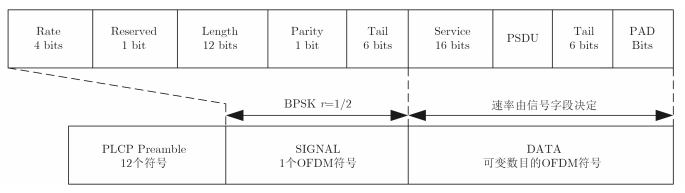图 1 OFDM模式物理层帧结构 Fig. 1 Physical layer frame structure of OFDM mode

OFDM模式下，基带复信号s(t)可以表示为：

 $S(t)\! = {S_{\rm{preamble}}}(t) \!+ \!{S_{\rm{signal}}}(t \!-\! {T_{\rm{pre}}})\! + \!{S_{\rm{data}}}(t \!- \!{T_\rm{d}})$ (1)

3 参考信号获取方法 3.1 参考通道信号模型

 ${y_{\rm{ref}}}(t) = {A_{\rm{ref}}}s(t) + \sum\limits_{k = 1}^N {{\lambda _k}} s(t - {\tau _k}) + {n_{\rm{ref}}}(t)$ (2)

3.2 基于同步信号的自适应滤波提纯算法

 $\hat s(t) = \sum\limits_{k = 0}^{M - 1} {{w_k}} x(n - k)$ (3)

3.3 基于WiFi发射信号的重构算法

OFDM技术有很强的抗多径衰落和窄带噪声的能力，参考信号提取应该充分利用信号结构本身的优势，为此，在深究WiFi信号形成机制的基础上，我们提出了适用于WiFi无源探测的参考信号重构方法，其流程如图 2所示。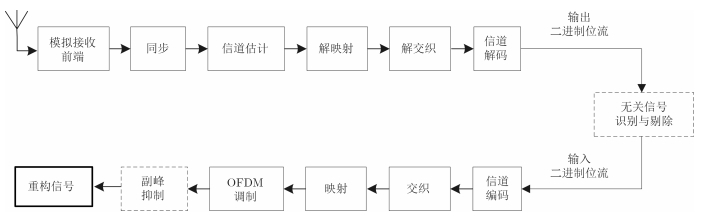图 2 WiFi信号重构流程框图 Fig. 2 Block diagram of WiFi signal reconstruction

3.3.1 同步算法

 $\quad \ \; {C_n} = \sum\limits_{k = 0}^{L - 1} {{r_{n + k}}} {{{\Large { \cdot } }}} \mathop r\nolimits_{n + k + D}^*$ (4)
 ${P_n} = \sum\limits_{k = 0}^{L - 1} {{r_{n + k + D}}} {{{\Large { \cdot } }}} \mathop r\nolimits_{n + k + D}^* = \sum\limits_{k = 0}^{L - 1} {{{\left| {{r_{n + k + D}}} \right|}^2}}$ (5)
 $\quad \ {M_n} = \sum\limits_{k = 0}^{L - 1} {{{\left| {{C_n}} \right|}^2}} /\mathop P\nolimits_n^2$ (6)

 $\begin{array}{*{20}{l}} {{\rm{Local}}\_{\rm{corr}}(n)}\\ { = \frac{{{{\left| {\sum\limits_{k = 0}^{L - 1} {r\left( {n + k} \right) \cdot {\rm{Local\_long}}*\left( k \right)} } \right|}^2}}}{{\sum\limits_{k = 0}^{L - 1} {{{\left| {r\left( {n + k} \right)} \right|}^2} \cdot \sum\limits_{k = 0}^{L - 1} {{{\left| {{\rm{Local\_long}}\left( k \right)} \right|}^2}} } }}} \end{array}$ (7)

 $\quad \quad \quad \quad \quad \ \ \,R = \sum\limits_{k = 0}^{L - 1} {{r_n}} {{{\Large { \cdot } }}} \mathop r\nolimits_{n + D}^*$ (8)
 $\Delta f = - \angle R/2{{π}} D{T_\rm{{sym}}}$ (9)

3.3.2 信道估计和均衡

 ${\Phi _{m,k}} = \arg \left[ {\sum\limits_{k = 1}^{{k_p}} {{R_{n,k}}{{({{\hat H}_{n,k}} \cdot {P_{n,k}})}^*}} } \right] = 2\pi n\Delta f{T_{sym}}$ (10)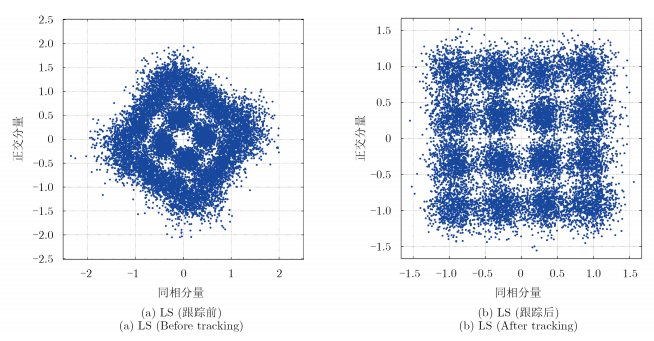图 3 基于LS信道估计均衡图 Fig. 3 Channel estimation equalization based on LS

3.3.3 参考信号修正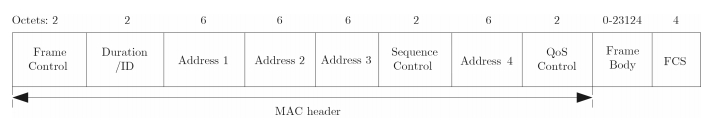图 4 MAC帧结构 Fig. 4 MAC frame structure

4 参考信号获取算法分析与比较 4.1 重构质量与误比特率关系分析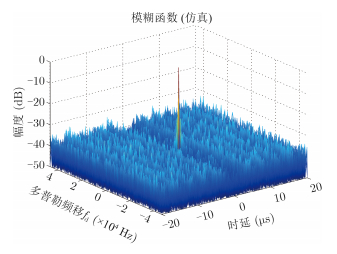图 5 重构信号与发送信号互相关函数图 Fig. 5 Mutual correlation function of the reconstructed signal and the transmitted signal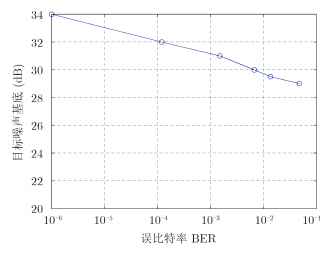图 6 误比特率与互相关函数噪声基底之间的关系图 Fig. 6 The relationship between the bit error rate and the cross correlation function noise base
4.2 提纯性能比较

 $\eta = \frac{{\sum\limits_{i = 1}^N {{{\left| {s\left( i \right) - {s_{\rm{o}}}\left( i \right)} \right|}^2}} }}{{\sum\limits_{i = 1}^N {{{\left| {{s_{\rm{o}}}\left( i \right)} \right|}^2}} }} \times 100\%$ (11)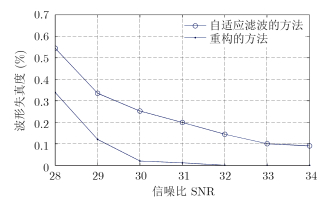图 7 不同直达波提取方法的波形失真度 Fig. 7 Waveform distortion of different direct wave extraction methods

5 重构质量对探测性能的影响分析

5.1 参考信号质量对时域杂波抑制方法的影响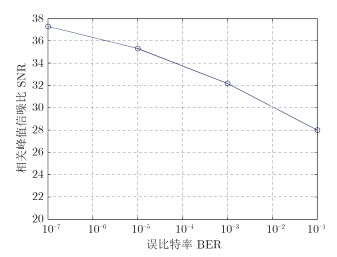图 8 参考信号重构时不同误码率的相关峰值信噪比 Fig. 8 Correlation peak signal to noise ratio of different bit error rate when the reference signal is reconstructed

5.2 实测信号处理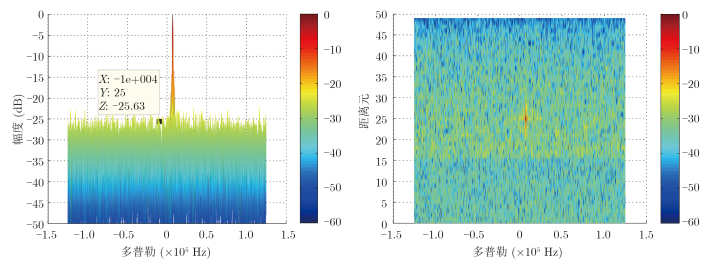图 9 原始发送信号的目标相关图，时延-幅度(左)，时延-多普勒(右) Fig. 9 The original transmission signal of the target correlation graph, delay-amplitude (left), delay-Doppler (right)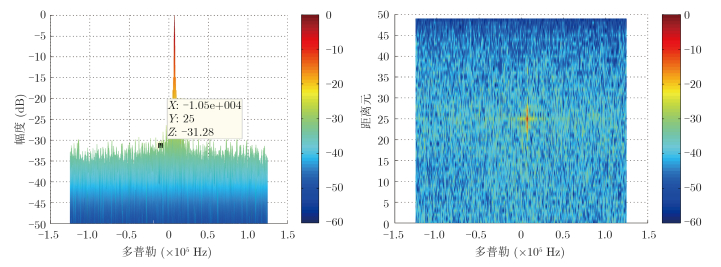图 10 重构信号的目标相关图，时延-幅度(左)，时延-多普勒(右) Fig. 10 Target correlation figure of the reconstructed signal, delay-amplitude (left), delay-Doppler (right)
6 结论

  Griffiths H D. New direction in bistatic radar[C]. IEEE RADAR Conference, Rome, 2008: 1-6.(1)  万显荣, 易建新, 程丰, 等. 单频网分布式外辐射源雷达技术[J]. 雷达学报, 2014, 3(6): 623-631. Wan Xian-rong, Yi Jian-xin, Cheng Feng, et al.. Single frequency network based distributed passive radar technology[J]. Journal of Radars, 2014, 3(6): 623-631.(3)  Chen Q, Tan B, Woodbridge K, et al.. Indoor target tracking using high Doppler resolution passive Wi-Fi radar[C]. 2015 IEEE International Conference on Acoustics, Speech and Signal Processing (ICASSP), South Brisbane, QLD, 2015: 5565-5569.(4)  Falcone P, Colone F, Macera A, et al.. Two-dimensional location of moving targets within local areas using WiFi-based multistatic passive radar[J]. IET, Radar, Sonar & Navigation, 2014, 8(2): 123-131.(3)  Colone F, Pastina D, Falcone P, et al.. WiFi-based passive ISAR for high-resolution cross-range profiling of moving targets[J]. IEEE Transactions on Geoscience and Remote Sensing, 2014, 52(6): 3486-3501.(3)  Falcone P, Bongioanni C, and Lombardo P. WiFi-based passive bistatic radar: data processing schemes and experimental results[J]. IEEE Transactions on Aerospace and Electronic Systems, 2012, 48(2): 1061-1079.(2)  Colone F, Woodbridge K, Guo H, et al.. Ambiguity function analysis of wireless lan transmissions for passive radar[J]. IEEE Transactions on Aerospace and Electronic Systems, 2011, 47(1): 240-264.(2)  Chetty K, Smith G E, and Woodbridge K. Through-The-Wall sensing of personnel using passive bistatic WiFi radar at standoff distances[J]. IEEE Transactions on Geoscience and Remote Sensing, 2012, 50(4): 1218-1226.(2)  饶云华, 朱逢园, 张修志, 等. WiFi 外辐射源雷达信号模糊函数及副峰抑制分析[J]. 雷达学报, 2012, 1(3): 225-231. Rao Yun-hua, Zhu Feng-yuan, Zhang Xiu-zhi, et al.. Ambiguity function analysis and side peaks suppression of passive radar[J]. Journal of Radars, 2012, 1(3): 225-231.(1)  万显荣, 唐慧, 王俊芳, 等. DTMB外辐射源雷达参考信号纯度对探测性能的影响分析[J]. 系统工程与电子技术, 2013, 35(4): 725-729. WAN Xian-rong, Tang Hui, Wang Jun-fang, et al.. Influence of reference signal purity on target detection performance in DTMB-based passive radar[J]. Systems Engineering and Electronics, 2013, 35(4): 725-729.(1)  Mazhar H and Hassan S A. Analysis of target multipaths in WiFi-based passive radars[J]. IET, Radar, Sonar & Navigation, 2016, 10(1): 140-145.(1)  吴海洲, 陶然, 单涛. 数字电视辐射源雷达基于空域滤波的直达波获取[J]. 兵工学报, 2009, 30(2): 226-230. Wu Hai-zhou, Tao Ran, and Shan Tao. Direct-path signal obtaining to digital video broadcasting transmitter radar based on the spatial filtering[J]. Acta Armamentarii, 2009, 30(2): 226-230.(1)  万显荣, 岑博, 易建新, 等. 中国移动多媒体广播外辐射源雷达参考信号获取方法研究[J]. 电子与信息学报, 2012, 34(2): 338-343. Wan Xian-rong, Cen Bo, Yi Jian-xin, et al.. Reference signal extraction methods for CMMB-based passive bistatic radar[J]. Journal of Electronics & Information Technology, 2012, 34(2): 338-343.(2)  Harms H A, Davis L M, and Palmer J. Understanding the signal structure in DVB-T signals for passive radar detection[C]. IEEE Radar Conference, Washington, 2010: 532-537.  Chetty K, Smith G, Guo H, et al.. Target detection in high clutter using passive bistatic WiFi radar[C]. IEEE Radar Conference, Pasadena, 2009: 1-5.  IEEE std 802.11g-2003. Telecommunications and information exchange between systems local and metropolitan area networks specific requirements Part 11: Wireless LAN Medium Access Control (MAC) and Physical Layer (PHY) specifications-Amendment 4: Further Higher-Speed Physical Layer Extension in the 2.4 GHz Band[S]. New York: Institue of Electrical and Electronics Engineers, Inc., 2003.(1)  Nasraoui L, Atallah L N, and Siala M. An efficient reduced-complexity two-stage differential sliding correlation approach for OFDM synchronization in the multipath channel[C]. 2012 IEEE Wireless Communications and Networking Conference (WCNC), 2012: 2059-2063.(1)